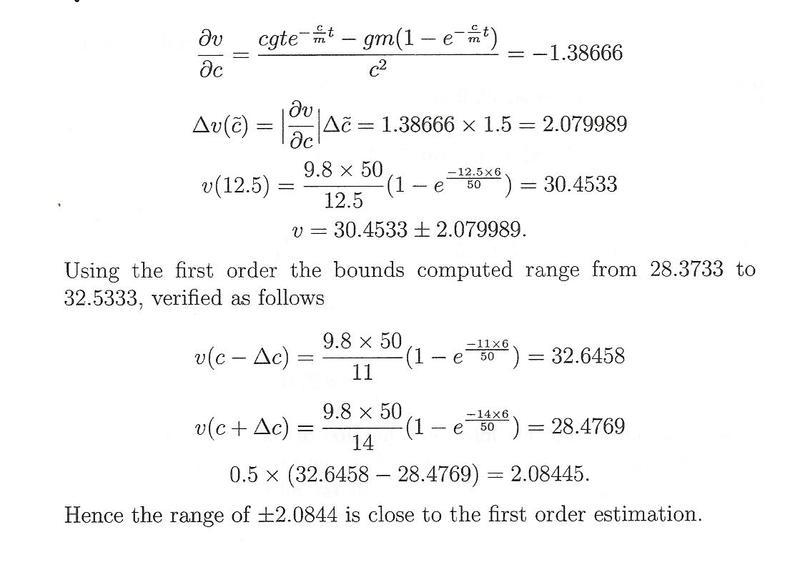# First Order Error Analysis (Taylor Series)

• jegues
So, to estimate the error, you take the derivative of the function and multiply it by the difference in the variable, in this case t, and the uncertainty in the variable, which is 1.5 for c. Then you plug in the values for t and c, and that gives you the error. So, in summary, the error of the velocity at t = 6 can be estimated using first-order error analysis by taking the derivative of the given function and multiplying it by the difference in the variable t and the uncertainty in the variable c, and then plugging in the values for t and c.

## Homework Statement

The equation for the velocity of a falling parachutist can be computed by,

$$v(t) = \frac{gm}{c}(1-e^{-(\frac{c}{m})t})$$

Use a first-order error analysis to estimate the error of v at t = 6, if g = 9.8 and m = 9.8, c = 12.5 plus or minus 1.5.

## The Attempt at a Solution

I've never done "first order error analysis" using taylor series so I've looked at the solution to try and do the problem backwards but I can't make sense of what's going on. (See figure attached)Can someone clarify to me what they're doing here? I need the ideas behind what's being done.

Thanks again!

#### Attachments

He is using approximation by differentials. In one variable, say you have a function f(x) and you know it and its derivative at x0. You can approximate its value at some nearby point x1 by

f(x1) ≈ f(x0) + f'(x0)(x1-x0)

You may see it written as

f(x1) - f(x0) ≈ f'(x0)(x1-x0)

or

Δy ≈ f'(x)Δx

For example, the second line in your image is exactly this last equation with y and x replaced by v and c.

Last edited:
LCKurtz said:
He is using approximation by differentials. In one variable, say you have a function f(x) and you know it and its derivative at x0. You can approximate its value at some nearby point x1 by

f(x1) ≈ f(x0) + f'(x0)(x1-x0)

You may see it written as

f(x1) - f(x0) ≈ f'(x0)(x1-x0)

or

Δy = f'(x)Δx

For example, the second line in your image is exactly this last equation with y and x replaced by v and c.

EDIT: I think I got it now.